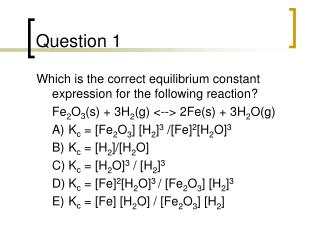DownloadDownload PresentationQuestion 1

# Question 1

Télécharger la présentation## Question 1

- - - - - - - - - - - - - - - - - - - - - - - - - - - E N D - - - - - - - - - - - - - - - - - - - - - - - - - - -
##### Presentation Transcript

1. Question 1 • Which is the correct equilibrium constant expression for the following reaction? • Fe2O3(s) + 3H2(g) <--> 2Fe(s) + 3H2O(g) • A) Kc = [Fe2O3] [H2]3 /[Fe]2[H2O]3 • B) Kc = [H2]/[H2O] • C) Kc = [H2O]3 / [H2]3 • D) Kc = [Fe]2[H2O]3 / [Fe2O3] [H2]3 • E) Kc = [Fe] [H2O] / [Fe2O3] [H2]

2. Question 2 • The equilibrium constant expression for the reaction 2BrF5(g) <--> Br2(g) + 5F2(g) is • A) Kc =[Br2] [F2]/[BrF5] • B) Kc = [Br2] [F2]5 /[BrF5]2 • C) Kc = [Br2] [F2]2 /[BrF5]5 • D) Kc = [BrF5]2 /[Br2][F2]5 • E) Kc = 2[BrF5]2 /([Br2] x 5[F2]5)

3. Question 3 • The following reactions occur at 500 K. Arrange them in order of increasing tendency to proceed to completion (least --> greatest tendency). • 1. 2NOCl <--> 2NO + Cl2 Kp = 1.7 x 10-2 • 2. 2SO3 <--> 2SO2 + O2 Kp = 1.3 x 10 -5 • 3. 2NO2 <--> 2NO + O2 Kp = 5.9 x 10 -5 • A) 2<1<3 • B) 1<2<3 • C) 2<3<1 • D) 3<2<1 • E) 3<1<2

4. Question 7 • Which of the following is a true statement about chemical equilibria in general? • A) At equilibrium the total concentration of products equals the total concentration of reactants, that is, [products] = [reactants]. • B) Equilibrium is the result of the cessation of all chemical change. • C) There is only one set of equilibrium concentrations that equals the Kc value. • D) At equilibrium, the rate constant of the forward reaction is equal to the rate constant for the reverse reaction. • E) At equilibrium, the rate of the forward reaction is equal to as the rate of the reverse reaction.

5. Question 11 • On analysis, an equilibrium mixture for the reaction 2H2S(g) <--> 2H2(g) + S2(g) was found to contain 1.0 mol H2S, 4.0 mol H2, and 0.80 mol S2 in a 4.0 L vessel. Calculate the equilibrium constant, Kc, for this reaction. • A) 1.6 • B) 3.2 • C) 12.8 • D) 0.64 • E) 0.8

6. Question 15 • The brown gas NO2 and the colorless gas N2O4 exist in equilibrium, 2NO2 <--> N2O4. In an experiment, 0.625 mole of N2O4 was introduced into a 5.00 L vessel and was allowed to decompose until equilibrium was reached. The concentration of N2O4 at equilibrium was 0.0750 M. Calculate Kc for the reaction. • A) 7.5 • B) 0.125 • C) 0.0750 • D) 0.10 • E) 0.050

7. Question 16 • Calculate Kc for the reaction 2HI(g) <--> H2(g) + I2(g) given that the concentrations of • each species at equilibrium are as follows: • [HI] = 0.85 mol/L, [I2] = 0.60 mol/L, [H2] = 0.27 mol/L. • A) 5.25 • B) 0.22 • C) 4.5 • D) 0.19 • E) 1.6 x 102

8. C B C C D A E C E D B A D C A B B E A D Answer Key – Chapter 14